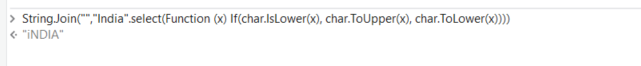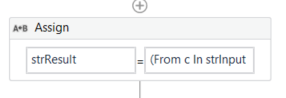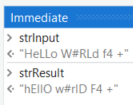# HOW write IndIA into iNDia

HOW write IndIA into iNDia

Could you Check the below expression :

``````varStr = "IndIA"
revCaseStr = String.Join("",varStr.Select(Function(x)if(Convert.ToByte(x)>=97 AndAlso Convert.ToByte(x)<=122,x.ToString.ToUpper,if(Convert.ToByte(x)>=65 AndAlso Convert.ToByte(x)<=90,x.ToString.ToLower,x.ToString))).ToArray)
``````

Here, `varStr` and `revCaseStr` are both variables of String type.

Let us know if this doesn’t work.

1 Like3 Likes

Combining with some Ideas from @supermanPunch we can do following to handle also non letter chars as following:(From c In strInput
Let chkL = Char.IsLetter(c)
Let cChg = If( chkL, If(Char.IsLower(c), Char.ToUpper(c), Char.ToLower(c)), c)
Select x =cChg.toString).Aggregate(Function (a,b) String.Join(“”,{a,b}))or simpified when splitted to 2 activities:

arrCharChanged | Array(of Char)=

``````(From c In strInput
Let chkL = Char.IsLetter(c)
Let cChg = If( chkL, If(Char.IsLower(c), Char.ToUpper(c), Char.ToLower(c)), c)
Select x =cChg).toArray
``````

strResult = String.Join(“”,arrCharChanged )